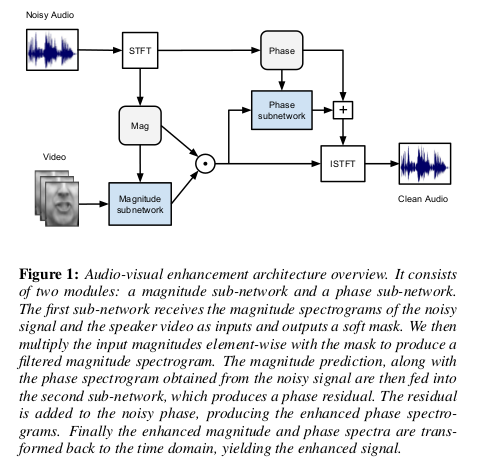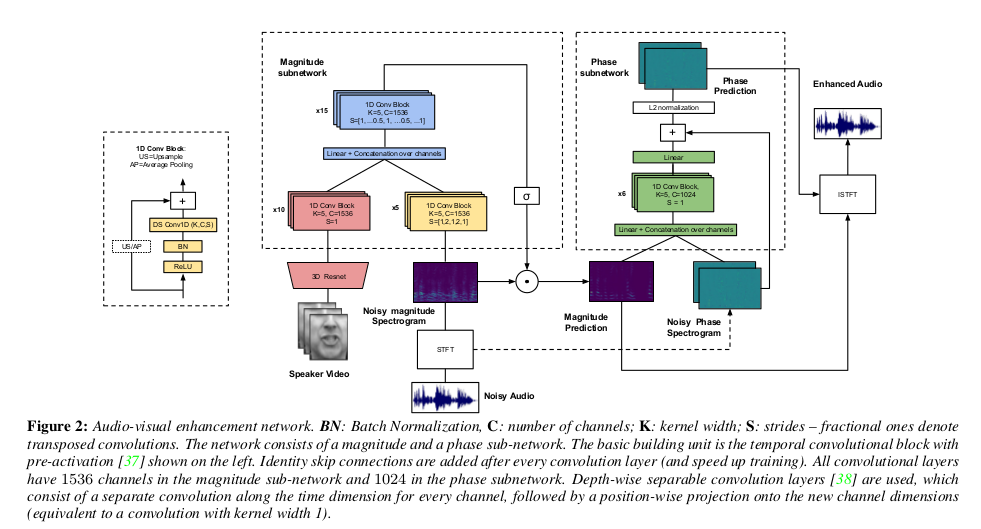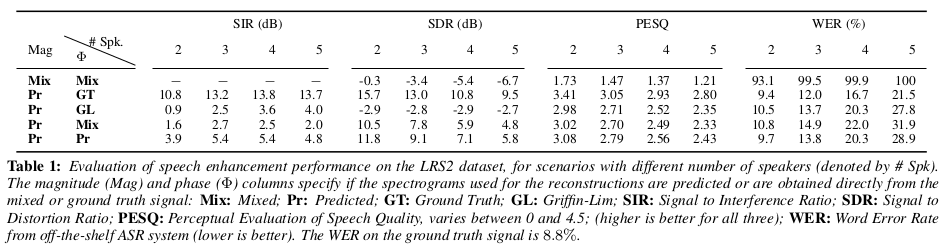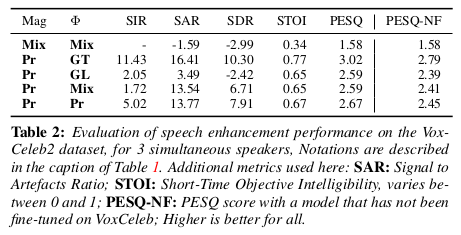# 论文引入

The Conversation：Deep Audio-Visual Speech Enhancement一文通过深度神经网络实现在多位发言者中分离出其中一位发言者的音频，为了进一步 展示我们看一下实验实现的视频结果（视频仅有YouTube资源，国内打开下面视频需要翻墙）。

• 不将频谱图视为图像，而是将频率区域视为信道的时间信号，这样能够构建一个具有大量参数的更深层网络，这些参数可以快速训练
• 生成一个用于过滤的软掩模，而不是直接预测幅度
• 设计了相位增强子网络
• 在视频演示了很好的实验效果

# 模型框架$\hat{M} = \delta (W_m^T f_{15}^{av}) \odot M_n$

$\phi_6 = \underbrace{ConvBlock(...ConvBlock([W_{m \phi }^T \hat{M};W_{n \phi }^T \Phi_n]))}_{\times 6}$ $\hat{\Phi} = \frac{W_{\phi}^T \phi_6 + \Phi_n}{\Vert \phi^T \phi_6 + \Phi_n) \Vert_2}$

$\mathcal L = \Vert \hat{M} - M^* \Vert - \lambda \frac{1}{TF} \sum_{t,f} M_{t,f}^* <\hat{\Phi_{t,f}},\Phi_{t,f}^*>$

# 实验